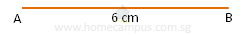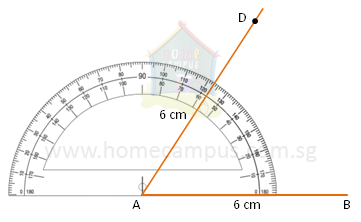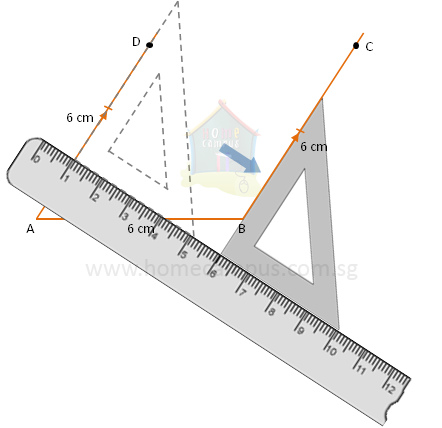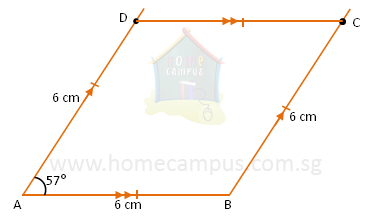## How to Draw a Rhombus?

Practice Unlimited Questions

#### 1. Draw a rhombus ABCD so that AB = 6 cm and ∠DAB = 57°.

Step 1: Draw a straight line AB 6 cm long.Step 2: Using a protractor draw an angle of 57° from Point A. Mark the Point D so that AD = 6 cm.All sides of a rhombus are equal.
Step 3: Using a ruler and a set square draw a line BC so that it is parallel to AD. Mark the Point C so that BC = 6 cm.Step 4: Join Point D to Point C.ABCD is the required rhombus.